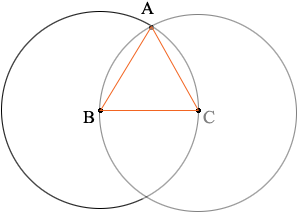SEARCH HOMEMath Central Quandaries & QueriesQuestion from Raraa, a student: There are two identical circles . The edge of one circle is at the middle point of the other circle. There were overlapped . The area of the overlapped surface is 20000 square centimetres . How do I find the radius of the circle rounded to the nearest whole centimetre ?Raraa,

I can help get you started.

Suppose the radius of each circle is r centimetres. Draw a diagram.What do you know about the triangle ABC?
What is the measure of the angle ABC?
What is the area of the left circle?
The sector ABC is a fraction of the left circle. What fraction is this?
What is the area of the sector ABC?
What is the area of the triangle ABC?

I hope this helps,
PennyMath Central is supported by the University of Regina and The Pacific Institute for the Mathematical Sciences.